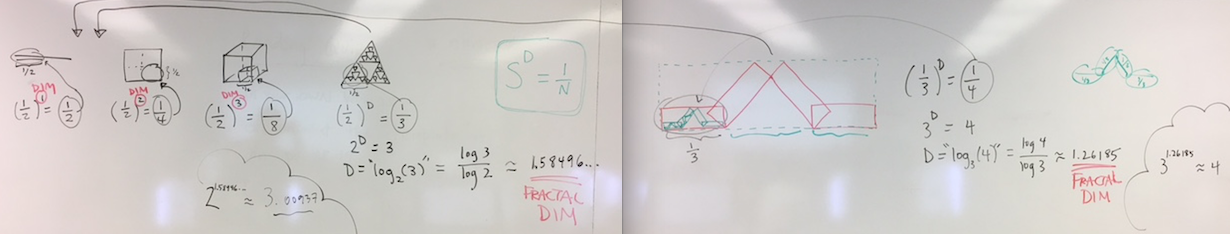# Thurs Sept 21 – Fractal dimension

Today we’ll work on finishing up the math for your blog posts; here’s the rundown:

• Help with length/area/volume calculations and fractal post (see my comments)
• Fractal dimension with the 3Blue1Brown fractal dimension video
• We’ll review some of what we watched last time (starting at 4:30),
• then continue watching (from 7:40),
• then stop (at time 9:40).

• Takeaways from that video:
• (scale-down factor)^(dimension) = 1/(number of copies)
• S^D = 1/N
• D = log_S (1/N)
• D = (ln S)/(ln 1/N)
• Picture of the board:• Can you calculate fractal dimension of your fractal? If not, calculate the fractal dimension of your “First Fractal” model instead.

### Finishing the Tinkercad Fractal post

• Change the name of “Initial Calculations” to “Volume Calculations” (or length, or area, as appropriate).
• Add a final section called “Fractal Dimension” to your blog post, and include your calculation of fractal dimension. Explain your work as much as possible.
• If you need to reprint your fractal, or to print it larger, then do that before the next class, and add a picture of the print to your post.
• Change your Tinkercad Fractal blog post Featured Image to the photo of your final 3D printed fractal.